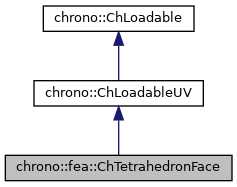chrono::fea::ChTetrahedronFace Class Reference

## Description

Face of a tetrahedron-shaped element.

The face is identified by the number of the vertex to which it is opposed: 0,1,2,3. Corner nodes, obtainable with GetNodeN(), are in counterclockwise order seen from the outside.

#include <ChTetrahedronFace.h>

Inheritance diagram for chrono::fea::ChTetrahedronFace:[legend]
Collaboration diagram for chrono::fea::ChTetrahedronFace:[legend]

## Public Member Functions

ChTetrahedronFace (std::shared_ptr< ChElementTetrahedron > element, char id)
Construct the specified face (0 <= id <= 3) on the given tetrahedral element.

std::shared_ptr< ChNodeFEAxyzGetNodeN (int i) const

void ShapeFunctions (ChVectorN< double, 3 > &N, double r, double s)
Fills the N shape function vector (size 3) with the values of shape functions at r,s 'area' coordinates, all ranging in [0...1].

Get the number of DOFs affected by this element (position part).

Get the number of DOFs affected by this element (speed part).

virtual void LoadableGetStateBlock_x (int block_offset, ChState &mD) override
Get all the DOFs packed in a single vector (position part).

virtual void LoadableGetStateBlock_w (int block_offset, ChStateDelta &mD) override
Get all the DOFs packed in a single vector (speed part).

virtual void LoadableStateIncrement (const unsigned int off_x, ChState &x_new, const ChState &x, const unsigned int off_v, const ChStateDelta &Dv) override
Increment all DOFs using a delta.

virtual int Get_field_ncoords () override
Number of coordinates in the interpolated field: here the {x,y,z} displacement.

virtual int GetSubBlocks () override
Get the number of DOFs sub-blocks.

virtual unsigned int GetSubBlockOffset (int nblock) override
Get the offset of the specified sub-block of DOFs in global vector.

virtual unsigned int GetSubBlockSize (int nblock) override
Get the size of the specified sub-block of DOFs in global vector.

virtual bool IsSubBlockActive (int nblock) const override
Check if the specified sub-block of DOFs is active.

virtual void LoadableGetVariables (std::vector< ChVariables * > &mvars) override
Get the pointers to the contained ChVariables, appending to the mvars vector.

virtual void ComputeNF (const double U, const double V, ChVectorDynamic<> &Qi, double &detJ, const ChVectorDynamic<> &F, ChVectorDynamic<> *state_x, ChVectorDynamic<> *state_w) override
Evaluate N'*F , where N is some type of shape function evaluated at U,V coordinates of the surface, each ranging in 0..+1 F is a load, N'*F is the resulting generalized load. More...

virtual bool IsTriangleIntegrationNeeded () override
If true, use quadrature over u,v in [0..1] range as triangle volumetric coords.

virtual ChVector ComputeNormal (const double U, const double V) override
Get the normal to the surface at the parametric coordinate u,v. More...

## ◆ ComputeNF()

 virtual void chrono::fea::ChTetrahedronFace::ComputeNF ( const double U, const double V, ChVectorDynamic<> & Qi, double & detJ, const ChVectorDynamic<> & F, ChVectorDynamic<> * state_x, ChVectorDynamic<> * state_w )
inlineoverridevirtual

Evaluate N'*F , where N is some type of shape function evaluated at U,V coordinates of the surface, each ranging in 0..+1 F is a load, N'*F is the resulting generalized load.

Returns also det[J] with J=[dx/du,..], which may be useful in Gauss quadrature.

Parameters
 U parametric coordinate in surface V parametric coordinate in surface Qi result of N'*F , maybe with offset block_offset detJ det[J] F Input F vector, size is = n.field coords. state_x if != 0, update state (pos. part) to this, then evaluate Q state_w if != 0, update state (speed part) to this, then evaluate Q

## ◆ ComputeNormal()

 virtual ChVector chrono::fea::ChTetrahedronFace::ComputeNormal ( const double U, const double V )
inlineoverridevirtual

Get the normal to the surface at the parametric coordinate u,v.

Normal must be considered pointing outside in case the surface is a boundary to a volume.